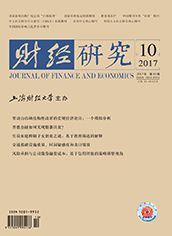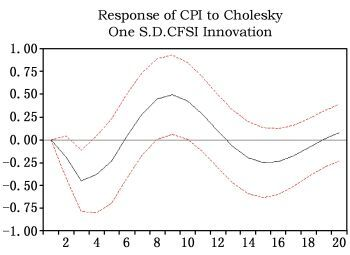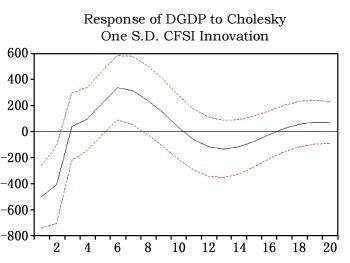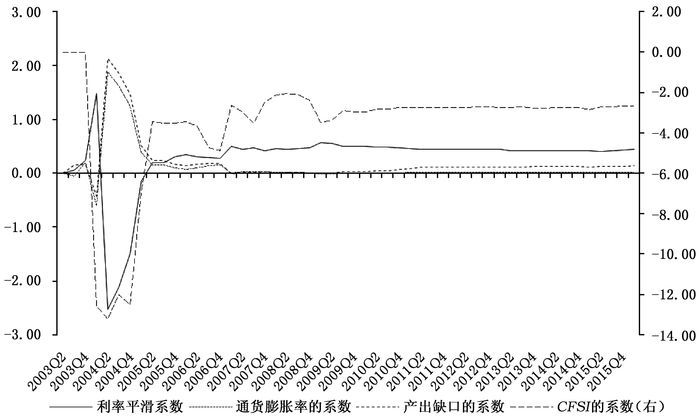﻿ 金融稳定目标下中国货币政策规则研究
 财经研究2017, Vol. 43Issue (10): 18-300

#### 文章信息财经研究2017年43卷第10期

Xu Guoxiang, Guo Jianna.

Research on chinese monetary policy rules under the target of financial stability

Journal of Finance and Economics, 2017, 43(10): 18-30.

### 文章历史《财经研究》
2017第43卷第10期

1. 上海财经大学 应用统计研究中心，上海 200433;
2. 上海财经大学 统计与管理学院，上海 200433

Research on Chinese Monetary Policy Rules under the Target of Financial Stability
Xu Guoxiang1,2, Guo Jianna21. Research Center for Applied Statistics, Shanghai University of Finance and Economics, Shanghai 200433, China;
2. School of Statistics and Management, Shanghai University of Finance and Economics, Shanghai 200433, China
Abstract: The monetary policy rules that do not take the financial stability target into account ignore the potential risk accumulation in the excesses financial prosperity under stable prices. On the basis of the two monetary policy target variables that are inflation and output respectively, it introduces financial stability in exponential form into the monetary policy targets and studies the characteristics of Taylor rule that includes financial stability target.The following empirical results have been found:firstly, financial stability and output can communicate smoothly in the monetary policy while inflation has no smooth transmission in the monetary policy; secondly, in the economic environment with high inflation, financial stability has a significant influence on interest rates, and the central bank has more focus on financial stability target; and at this state, the expansion of the output gap has greater effect on interest rates compared with the low inflation; furthermore, interest rates need to be more responsive to the deflation; thirdly, if monetary policy rule that does not include financial stability target is regarded as the reference rule of macroeconomic regulation, the effects of monetary policy regulation is underestimated; fourthly, in monetary policy regulation, China significantly focuses on financial stability target, and China's macro-control gradually tends to be adjusted in accordance with Taylor rule containing financial stability, so the research content and conclusions concerning the introduction of financial stability target into the monetary policy rules are effective.
Key words: financial stability index    monetary policy    Taylor rule

① 在线性模型中，不管经济环境如何，货币政策目标变量的增加或减少对利率的影响是相同的；而在非线性模型中，在不同的经济环境下，货币政策目标变量的增加或减少对利率的影响是不同的。

② 一般地，货币政策的非对称效应表现为，在经济紧缩阶段扩张性货币政策的加速作用小于在经济扩张阶段紧缩性货币政策的减速作用。

20世纪90年代至21世纪初，美联储、英格兰银行、日本银行、欧洲中央银行等的政策执行符合泰勒规则，这些国家迎来了经济和金融繁荣发展的一段时期。关于泰勒规则在我国的应用有三点一致的结论：第一，泰勒规则在全球范围内得到广泛认可和使用，对央行的货币操作具有重要的指导意义；第二，泰勒规则可以描述我国的利率走势，有一定的适用性；第三，泰勒规则在我国是不稳定的规则，主要是利率对通货膨胀的反应不稳定，应推进利率改革，促进货币政策操作的规则化。相关文献主要有谢平和罗雄(2002)、张屹山和张代强(2007)、刘金全和张小宇(2012)、袁野(2014) 等。鉴于此，本文使用泰勒规则来研究加入金融稳定目标的货币政策规则。

 $r_t^* = {r^*} + \beta \left( {E\left[ {{\pi _{t + i}}{\rm{|}}{\Omega _t}} \right] - \pi _{t + i}^*} \right) + \gamma E\left[ {{y_{t + j}}{\rm{|}}{\Omega _t}} \right]$ (1)

 ${r_t} = \rho {r_{t - 1}} + \left( {1 - \rho } \right)r_t^*$ (2)

 ${r_t} = \left( {1 - \rho } \right)\left[ {{r^*} + \beta \left( {{\pi _{t + i}} - \pi _{t + i}^*} \right) + \gamma {y_{t + j}}} \right] + \rho {r_{t - 1}}$ (3)

 ${r_t} = \left( {1 - \rho } \right)\left[ {{r^*} + \beta \left( {{\pi _{t + i}} - \pi _{t + i}^*} \right) + \gamma {y_{t + j}}} \right] + \delta cfs{i_{t + k}} + \rho {r_{t - 1}} + {\varepsilon _t}$ (4)

α0=(1－ρ)(r*-βπt+i*)，α1=(1－ρ)βα2=(1－ρ)γα3=δ，则式(4) 可写为：

 ${r_t} = {\alpha _0} + {\alpha _1}{\pi _{t + i}} + {\alpha _2}{y_{t + j}} + {\alpha _3}cfs{i_{t + k}} + \rho {r_{t - 1}} + {\varepsilon _t}$ (5)

 ${r_t} = \psi \prime {z_t} + \theta \prime {z_t}G\left( {\eta ,{\rm{ }}c,{\rm{ }}{s_t}} \right) + {\varepsilon _t},{\rm{ }}t = 1, \ldots ,{\rm{ }}T,{\varepsilon _t} \sim i.i.d.N\left( {0,{\rm{ }}{\sigma ^2}} \right)$ (6)

 $G\left( {\eta ,{\rm{ }}c,{\rm{ }}{s_t}} \right) = {\left( {1 + {\rm{exp}}\left\{ { - \eta \prod\limits_{k = 1}^K {\left( {{s_t} - {c_k}} \right)} } \right\}} \right)^{ - 1}},\eta > 0$ (7)

K=1，式(7) 为Logistic STRLSTR1模型；当K=2时，式(7) 为LSTR2模型。

 $\begin{array}{l} {r_t} = {\alpha _{0,{\rm{ }}t}} + {\alpha _{1,{\rm{ }}t}}{\pi _{t + i}} + {\alpha _{2,{\rm{ }}t}}{y_{t + j}} + {\alpha _{3,{\rm{ }}t}}cfs{i_{t + k}} + {\rho _t}{r_{t - 1}} + {\varepsilon _t}\\ {\alpha _{m,{\rm{ }}t}} = {\alpha _{m,{\rm{ }}t - 1}} + {\theta _{m,{\rm{ }}t}},{\rm{ }}{\theta _{m,{\rm{ }}t}} \sim i.i.d.N\left( {0,{\rm{ }}\sigma _{{\theta _m}}^2} \right),m = 0,{\rm{ }}1,{\rm{ }}2,{\rm{ }}3\\ {\rho _t} = {\rho _{t - 1}} + {\theta _{4,{\rm{ }}t}},{\theta _{4,{\rm{ }}t}} \sim i.i.d.N\left( {0,{\rm{ }}\sigma _{{\theta _4}}^2} \right) \end{array}$ (8)

(一) 中国金融稳定指数对通货膨胀和产出的预测能力

1.格兰杰因果检验。由表 1可知，CFSI是通货膨胀率的因，通货膨胀率不是CFSI的因，说明CFSI对通货膨胀有预测能力(戴国强和张建华，2009)。同样地，CFSI是产出的因，产出不是CFSI的因，说明CFSI对产出有预测能力。

 原假设 F统计值 P值 原假设 F统计值 P值 CPI不是CFSI的因 0.23049 0.8746 GDP不是CFSI的因 0.89225 0.9369 CFSI不是CPI的因 3.63565 0.0203 CFSI不是GDP的因 4.87311 0.0079 注：表 1、图 1和表 2中CPI表示通货膨胀率。本表是滞后期为3的检验结果，而滞后2期到滞后6期的结果都表明，CFSI分别是通货膨胀率和GDP的因，而通货膨胀率和GDP不是CFSI的因。因此，格兰杰因果检验的结果对滞后期不敏感。图 1 通货膨胀率对CFSI的脉冲响应

 被解释变量：CPI 领先期数 0 1 2 3 4 5 6 CFSI -22.39 -20.28 -13.78 -3.91 6.19 13.40 17.04 P值 0.0000 0.0000 0.0000 0.0000 0.0953 0.0001 0.0000 被解释变量：GDP 领先期数 0 1 2 3 4 5 6 CFSI -4089.32 765.26 5061.98 6919.90 7789.17 6738.06 4712.51 P值 0.0607 0.7303 0.0190 0.0010 0.0001 0.0013 0.0296

2.脉冲响应分析。我们分别建立CFSI与通货膨胀率、CFSIGDP的双变量VAR模型，结果见图 1图 2。可以看到，CFSI的一个冲击后，通货膨胀率在第3期达到最小值，在第9期达到最大值，GDP在第6期达到最大值。图 2 GDP对CFSI的脉冲响应

3.循环方程法的预测能力分析。我们使用循环方程法(Celine等，2004) 对CFSI的预测能力进行了分析。

 $CP{I_t} = \alpha + \beta CFS{I_{t - k}} + {\varepsilon _t},GD{P_t} = \alpha \prime + \beta \prime CFS{I_{t - k}} + \varepsilon {\prime _t}$ (9)

(二) 加入金融稳定目标的泰勒规则非线性特征

 $r = \left( {{r_1}{f_1} + {r_2}{f_2} + {r_3}{f_3}} \right)/\sum\limits_{i = 1}^3 {\left| {{f_i}} \right|}$ (10)

 ${\rm{产出缺口 = }}\left( {{\rm{实际产出}} - {\rm{潜在产出}}} \right)/{\rm{潜在产出}} \times 100\%$ (11)

 模型1 模型2 模型3 估计值 P值 估计值 P值 估计值 P值 线性部分 cons 0.8603 0.0002 1.2264 0.0002 1.7263 0.0032 r(t-1) 0.5864 0.0000 0.4722 0.0005 0.2884 0.0408 π(t) 0.0137 0.0353 0.0180 0.0228 0.0333 0.3320 y(t) 0.1742 0.0141 0.1609 0.0112 0.0207 0.8167 cfsi(t) -1.8781 0.0946 -3.5494 0.1183 非线性部分 cons -1.1913 0.4369 π(t) 0.0170 0.6930 y(t) 0.4288 0.0681 cfsi(t) 1.4597 0.6975 Gamma 10.0597 0.5034 C1 22.0121 0.0000 Adj. R2 0.7512 0.7727 0.8531 模型4 模型5 模型6 估计值 P值 估计值 P值 估计值 P值 线性部分 cons 4.8999 0.0659 1.1784 0.0002 1.0748 0.0004 r(t-1) 0.2082 0.1783 0.4847 0.0003 0.3288 0.0137 π(t) -0.0072 0.9484 0.0172 0.0309 0.0736 0.0059 y(t) -0.6630 0.0284 0.1682 0.0145 0.1254 0.0679 cfsi(t-1) -9.9720 0.1488 -1.4754 0.0801 非线性部分 cons -5.1370 0.1867 -0.1628 0.7966 π(t) 0.0804 0.5685 -0.0357 0.1595 y(t) 1.2504 0.0093 0.2682 0.0312 cfsi(t-1) 9.2376 0.3028 -2.3469 0.0891 Gamma 1.9282 0.0738 15.4711 0.3890 C1 11.1765 0.7827 20.6617 0.0000 C2 11.1765 0.7827 Adj. R2 0.8575 0.7555 0.8471 注：模型1的回归方程为rt=α0+α1πt+α2yt+ρrt－1+εt，工具变量为r(t-1)、π(t-1) 和y(t-1)。模型2的回归方程为rt=α0+α1πt+α2yt+α3cfsit+ρrt－1+εt，工具变量为r(t-1)、π(t-1)、y(t-1) 和cfsi(t－1)。模型3为LSTR1模型，将cfsi(t)引入线性及非线性部分。模型4为LSTR2模型，将cfsi(t-1) 引入线性及非线性部分。模型5的回归方程为rt=α0+α1πt+α2yt+α3cfsit-1+ρrt－1+εt，工具变量为r(t-1)、π(t-1)、y(t-1) 和cfsi(t－1)。模型6为LSTR1模型，将cfsi(t-1) 引入非线性部分。Gamma为STR中转换函数的斜率参数，C1和C2为位置参数。模型1、模型2和模型5使用GMM估计法。模型3、模型4和模型6中转换变量为π(t)，不考虑利率平滑效应随转换变量的变化而变化，使用网格搜索法寻找初始值。模型2可作为模型3的对比模型，模型5可作为模型6的对比模型。

k的取值在实证中确定。

① 此处，模型3指其线性部分。

② 受篇幅所限，模型结果未列入表 4。

③ 通货膨胀率以2003年第一季度为基期，以此阈值为标准，下文中高通胀指通货膨胀率高于此值，低通胀指通货膨胀率低于此值。图 3 模型6中通货膨胀率、产出缺口和CFSI的系数

(三) 加入金融稳定目标的泰勒规则时变特征

① 一般地，取i=j=k=0。

 $r = c\left( 1 \right) + p1 \times r\left( { - 1} \right) + p2 \times \pi + p3 \times y + p4 \times cfsi + \left[ {var = exp\left( {c\left( 2 \right)} \right)} \right]$ (12)

 $p1 = p1\left( { - 1} \right),p2 = p2\left( { - 1} \right),p3 = p3\left( { - 1} \right),p4 = p4\left( { - 1} \right)$ (13)图 4 状态空间模型中各变量的时变系数

① 受篇幅限制，估计结果未列出，如有需要可联系作者。

CFSI的系数依然为负，其绝对值经历了从较大值逐渐下降并趋于稳定的过程。从中可以看出，金融稳定对利率的影响逐渐平稳化，近年来稳定在-2.7附近。通货膨胀的系数大部分依然大于0且小于1，说明一直以来名义利率对通胀的反应不足。产出缺口的系数基本大于0，从长期来看，产出缺口的时变系数具有缓慢上升的趋势，表明相对于通货膨胀，央行更倾向于关注产出，这与图 3的结果一致。

  卞志村, 孙慧智, 曹媛媛. 金融形势指数与货币政策反应函数在中国的实证检验[J]. 金融研究, 2012(8): 44–55.  戴国强, 张建华. 中国金融状况指数对货币政策传导作用研究[J]. 财经研究, 2009(7): 52–62.  刁节文, 章虎. 基于金融形势指数对我国货币政策效果非线性的实证研究[J]. 金融研究, 2012(4): 32–44.  刁节文, 章虎, 李木子. 中国金融形势指数及其在货币政策中的检验[J]. 山西财经大学学报, 2011(7): 49–56.  封北麟, 王贵民. 金融状况指数FCI与货币政策反应函数经验研究[J]. 财经研究, 2006(12): 53–64. DOI:10.3969/j.issn.1001-9952.2006.12.006  高洁超, 孟士清. 中国非线性审慎利率规则的实证研究[J]. 南京审计学院学报, 2015(6): 95–104.  郭红兵, 杜金岷. 中国货币政策关注金融稳定吗?——纳入FSCI的货币政策反应函数的实证检验[J]. 广东财经大学学报, 2014(5): 4–13.  黄佳, 朱建武. 基于金融稳定的货币政策框架修正研究[J]. 财经研究, 2007(4): 96–106.  刘金全, 张小宇. 时变参数"泰勒规则"在我国货币政策操作中的实证研究[J]. 管理世界, 2012(7): 20–28.  马勇. 基于金融稳定的货币政策框架:理论与实证分析[J]. 国际金融研究, 2013(11): 4–15.  马勇. 金融稳定与宏观审慎理论框架及在中国的应用[M]. 北京: 中国金融出版社, 2016.  米尔顿·弗里德曼, 安娜·雅各布森·施瓦茨. 美国货币史: 1967-1960[M]. 巴曙松等, 译. 北京: 北京大学出版社, 2009.  万光彩, 张霆, 卫松涛. 基于金融稳定视角的货币政策与宏观审慎政策演进[J]. 东北农业大学学报(社会科学版), 2015(5): 31–37.  肖本华. 货币政策规则——行为经济学范式[M]. 上海: 上海财经大学出版社, 2012.  谢平, 罗雄. 泰勒规则及其在中国货币政策中的检验[J]. 经济研究, 2002(3): 3–12.  徐国祥, 郭建娜, 陈燃萍. 中国金融稳定指数的构建及其领先能力分析[J]. 统计与信息论坛, 2017(4): 3–9.  袁野. 时变参数的货币政策规则及其对利率期限结构的动态影响——基于资产价格波动、汇率因素对Taylor规则的修正[J]. 中央财经大学学报, 2014(5): 40–46.  张屹山, 张代强. 前瞻性货币政策反应函数在我国货币政策中的检验[J]. 经济研究, 2007(3): 20–32.  周波. 我国非线性货币政策规则研究[J]. 财经问题研究, 2014(1): 53–59.  Albulescu C T, Goyeau D, Pepin D. Financial instability and ECB monetary policy[J]. Economics Bulletin, 2013, 33(1): 388–400.  Baxa J, Horvath R, Vasicek B. Time-varying monetary policy rules and financial stress:Does financial instability matter for monetary policy?[J]. Journal of Financial Stability, 2013, 9(1): 117–138. DOI:10.1016/j.jfs.2011.10.002  Benjamin K. The Taylor rule and financial stability-A literature review with application for the Eurozone[J]. Magks Papers on Economics, 2014, 65(2): 159–192.  Bernanke B S. Asset-price "bubbles" and monetary policy[R]. Working Paper, 2002.  Bernanke B S, Mishkin F S. Inflation targeting:A new framework for monetary policy?[J]. Journal of Economic Perspectives, 1997, 11(2): 97–116. DOI:10.1257/jep.11.2.97  Castro V. Can central banks' monetary policy be described by a linear (augmented) Taylor rule or by a nonlinear rule?[J]. Journal of Financial Stability, 2011, 7(4): 228–246. DOI:10.1016/j.jfs.2010.06.002  Celine G, Graham C, Liu Y. Financial conditions indexes for Canada[R]. Bank of Canada Working Paper No.22, 2004.  Posen A S. Why central banks should not burst bubbles[J]. International Finance, 2006, 9(1): 109–124. DOI:10.1111/infi.2006.9.issue-1  Roubini N. Why central banks should burst bubbles[J]. International Finance, 2006, 9(1): 87–107. DOI:10.1111/infi.2006.9.issue-1  Schinasi G J. Responsibility of central banks for stability in financial markets[R]. IMF Working Paper WP/03/121, 2003.  Woodford M. Inflation targeting and financial stability[R]. NBER Working Paper No.17967, 2012.